4.1 Basic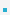Definition: A dynamic data structure that consists of a sequence of records where each element    contains a link to the next record in the sequence.Each record of a linked list is often called an element or node,
– Each node has a payload and a reference (i.e., a link) to the next and/or previous node in the    sequence.The start (head) of the list is maintained in a sequence variable.End of the list is indicated by NULL (sentinel).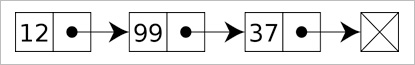Linear and circular lists
– If the last node points to the first node of the list, the list is said to be circular or circularly linked;   otherwise it is said to be open or linear.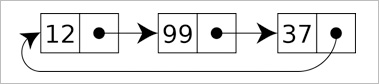Singly, doubly, and multiply linked lists
– Singly linked lists: Each node haa a data field as well as a next field, which points to the next node    in the linked list.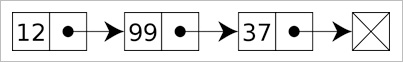– Doubly linked list: Each node contains, besides the next-node link, a second link field pointing to the    previous node in the sequence. The two links may be called forward(s) and backwards, or next and    prev(ious).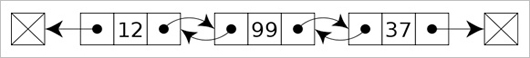A dynamic array is a data structure that allocates all elements contiguously in memory, and keeps a   count of the current number of elements.

 Name Linked lists Dynamic Array Size Dynamic Fixed Indexing O(n) O(1) Insert/Delete at beginning O(1) O(n) at end O(1) O(1) in middle search time + O(1) O(n)Operations
– insert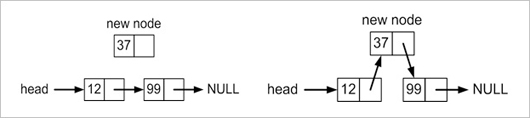– delete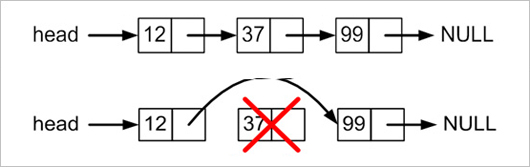Example

create_node()
 /* data strcture */ typedef struct node {          int data;             // payload          struct node *next; } node_type; /* creating new node */ node_type *create_node(int data) {      node_type *node = (node_type *)malloc(sizeof(node_type));      if (node!=NULL) {         node->data = data;         node->next = NULL;      }      return node; }

insert_node()
 /* insert after node */ node_type *insert_node(node_type **head, node_type *node, int data) {      node_type *newnode;      newnode = create_node(data);      if (newnode) {         /* insert newnode after node */         if (node) {            newnode->next = node->next;            node->next = newnode;         }         /* NULL list */         else {             newnode->next = NULL;             (*head) = newnode;         }     }     return newnode; }

delete_node()
 int delete_node(node_type **head, node_type *delnode) {     node_type *current = (*head);     if (!(*head)) return -1;     if (*head == delnode) { /* the node is the head */         *head = delnode->next;         free(delnode);         return 0;     }     else {         /* find the node to be deleted */         while (current->next && current->next!=delnode)             current = current->next;         if (current->next) {             current->next = delnode->next;             free(delnode);             return 0;         }     }     return -1; }

main()
 int main() {     int i;     node_type *head = NULL;     node_type *current, *delnode;     /* creat linked lists */     current = head;     for (i=0; i<10; i++) {         current = insert_node(&head, current, i);         if (i==3) delnode = current;     }     /* delete */     delete_node(&head, head);     delete_node(&head, delnode);     /* iterating */     for (current=head; current!=NULL; current=current->next)         printf("%d\n", current->data);     return 0; }If ptr points to any node in a doubly linked list, then:
ptr = ptr->prev->next = ptr->next->prevOperations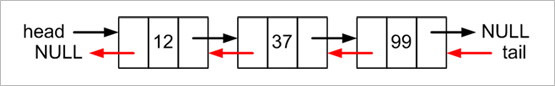Example

create_node()
 /* data strcture */ typedef struct node {     int data;           // payload     struct node *next;     struct node *prev; } node_type; /* creating new node */ node_type *create_node(int data) {     node_type *node = (node_type *)malloc(sizeof(node_type));     if (node!=NULL) {         node->data = data;         node->next = NULL;         node->prev = NULL;     }     return node; }

insert_node()
 node_type *insert_node(     node_type **head, node_type **tail, node_type *node, int data) {     node_type *newnode;     newnode = create_node(data);     if (newnode) {         /* insert newnode after node */         if (node) {             newnode->prev = node;             newnode->next = node->next;             if (node->next == NULL) (*tail) = newnode;             else node->next->prev = newnode;             node->next = newnode;         }         /* NULL list */        else {             newnode->prev = NULL;             newnode->next = NULL;             (*head) = newnode;             (*tail) = newnode;         }     }     return newnode; }

delete_node()
 int delete_node(node_type **head, node_type **tail, node_type *delnode) {     node_type *current = (*head);     if (!(*head) || !(delnode)) return -1;     /* the node to be deleted is the head */     if (*head == delnode) {         *head = delnode->next;         delnode->next->prev = NULL;     }     else if (*tail == delnode) {         *tail = delnode->prev;         delnode->prev->next = NULL;     }     else {         delnode->prev->next = delnode->next;         delnode->next->prev = delnode->prev;     }     free(delnode);     return 0; }

main()
 int main() {     int i;     node_type *head = NULL;     node_type *tail = NULL;     node_type *current, *delnode;      /* creat linked lists */     current = head;     for (i=0; i<10; i++) {         current = insert_node(&head, &tail, current, i);         if (i==3) delnode = current;     }     /* delete */     delete_node(&head, &tail, head);     delete_node(&head, &tail, tail);     delete_node(&head, &tail, delnode);      /* iterating */     for (current=head; current!=NULL; current=current->next)         printf("%d\n", current->data);     for (current=tail; current!=NULL; current=current->prev)         printf("%d\n", current->data);     return 0; }

 이전페이지 / 5 / 다음페이지